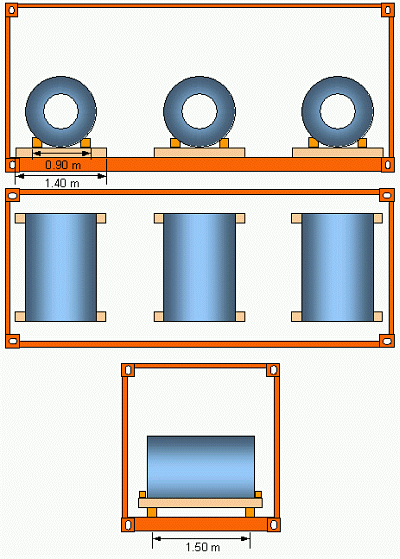6.2   Weight class L(max)In this example three coils of 9 t each shall be loaded into a container of P = 28.230 t. Thus, each coil has a mass of 31.9 % of the payload. The diameter is d = 1.10 m and the breadth is b = 1.66 m. The coils are tendered without skids. If the coils were stowed longitudinally using a C-hook, the longitudinal beams underneath would have a spread s = 0.75 m (Figure 24). Consequently, the beams needed to have a length of 2.60 m according to the diagram in Figure 13, in order not to overload the container bottom. But then only two of these coil could be loaded because 3 x 2.60 exceeds the length of the container.Figure 24: Narrow spread with a stowage "Eye fore and aft"

Thus the coils are stowed transversely into the container as shown in Figure 25 and arranged according to the rule (1/7, 1/2, 6/7), mentioned in chapter 3.2. This arrangement is symmetrical in longitudinal and transverse direction.Figure 25: Stowage of "light" coils with full payload (limit weight)Contact  |  Site Map  |  Glossary  |  Legal Notice